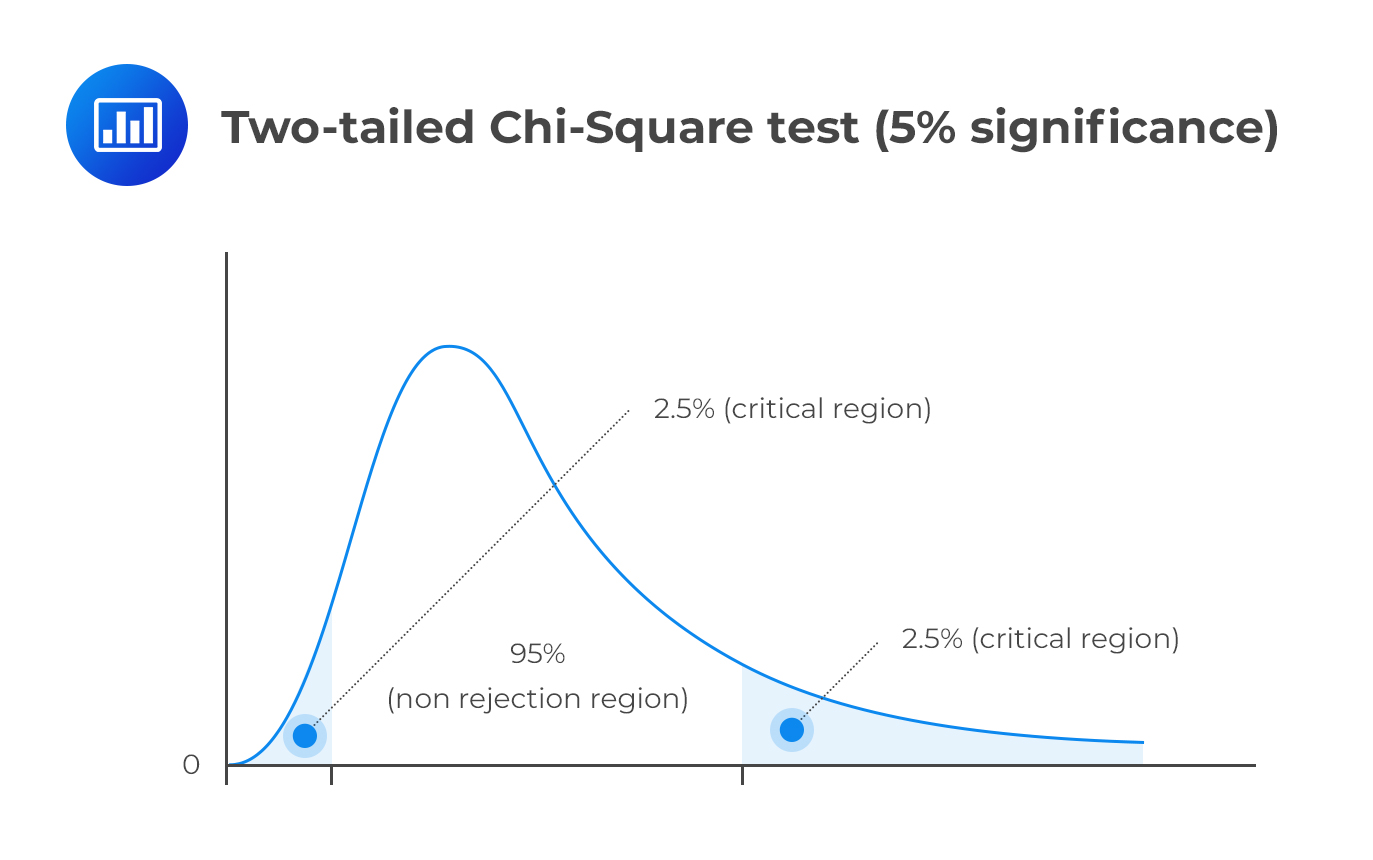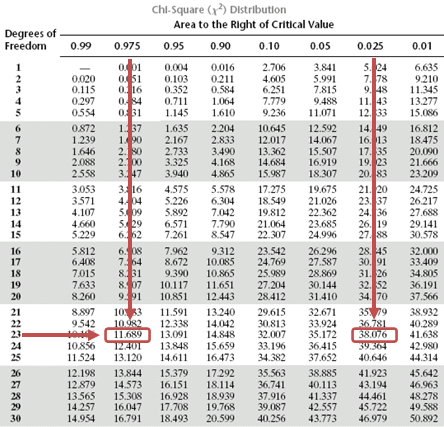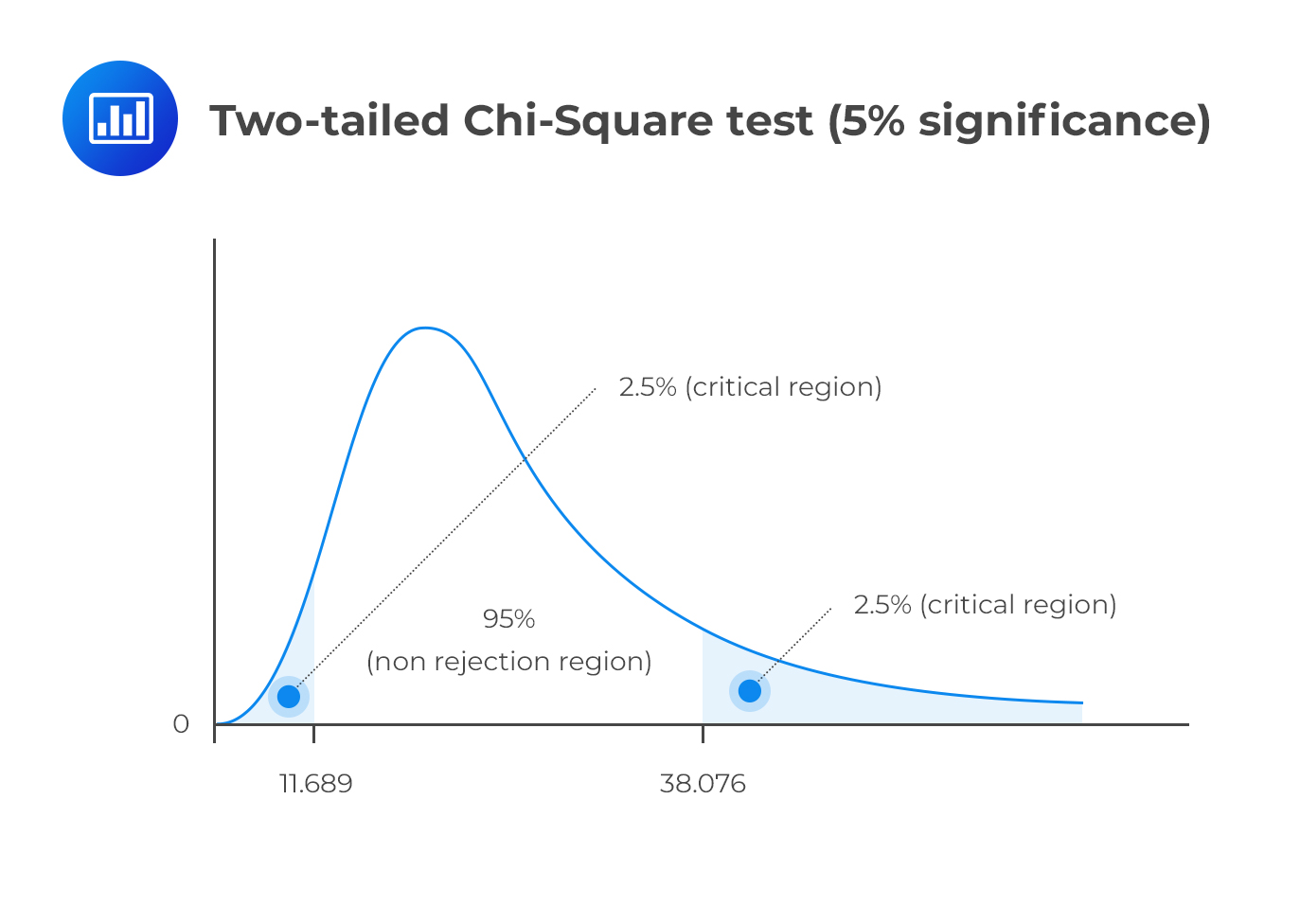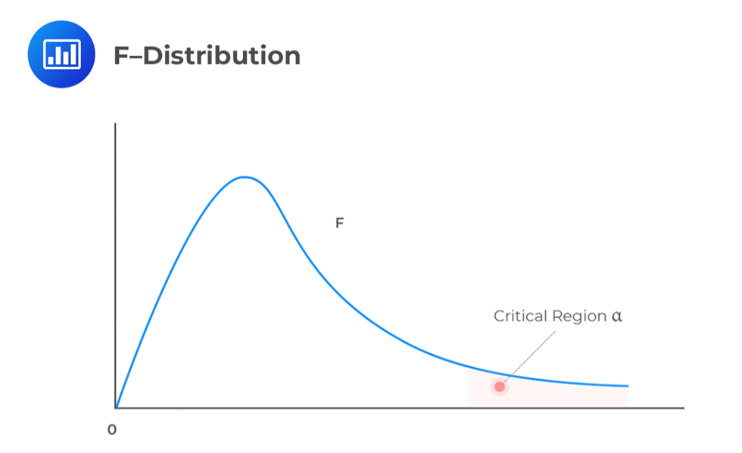Limited Time Offer: Save 10% on all 2022 Premium Study Packages with promo code: BLOG10# Chi-square Test of a Single Population Variance and F-test of the Ratio of Two Population Variances

## Testing the Variances of a Normally Distributed Population using the Chi-square Test

A chi-square test is used to establish whether a hypothesized value of variance is equal to, less than, or greater than the true population variance. Unlike most distributions covered in the CFA® Program curriculum, the chi-square distribution is asymmetrical. However, the distribution approaches the “normal” one as the degrees of freedom increase.As a natural consequence, the chi-square distribution has no negative values and is bounded by zero. A chi-square statistic with (n – 1) degrees of freedom is computed as:

$${ \chi }_{ n-1 }^{ 2 }=\frac { \left( n-1 \right) { S }^{ 2 } }{ { \sigma }_{ 0 }^{ 2 } }$$

Where:

$$n$$ = Sample size.

$$S^2$$ = Sample variance.

$${ \sigma }_{ 0 }^{ 2 }$$ = Hypothesized population variance.

### Example: Chi-square Test

For the 15-year period between 1995 and 2010, ABC’s monthly return had a standard deviation of 5%. John Matthew, CFA, wishes to establish whether the standard deviation witnessed during that period still adequately describes the long-term standard deviation of the company’s return. To achieve this end, he collects data on the monthly returns recorded between 1st January, 2015 and 31st December, 2016 and computes a monthly standard deviation of 4%.

Carry out a 5% test to determine if the standard deviation computed in the latter period is different from the 15-year value.

Solution

As is the norm, start by writing down the hypothesis

$$H_0: \sigma_0^2= 0.0025$$

$$H_1: \sigma^2 \neq 0.0025$$

Since the latter period has 24 months, n = 24. The test statistic is:

$${ \chi }_{ 24-1 }^{ 2 }=\frac { \left( 24-1 \right) { 0.0016 } }{ 0.0025 } =14.72$$

This is a two-tailed test. As such, we have to divide the significance level by two and screen our test statistic against the lower and upper 2.5% points of $${ \chi }_{ 23 }^{ 2 }$$.

Consulting the chi-square table, the test statistic (14.72) lies between the lower (11.689) and the upper (38.076) 2.5% points of the chi-square distribution.Therefore, we have insufficient evidence to reject the H0. It is, therefore, reasonable to conclude that the latter standard deviation value is close enough to the 15-year value.

## Testing the Equality of the Variances of Two Normally Distributed Populations based on Two Independent Random Samples using the F-distribution

Assume that we have 2 independent random samples of sizes n1 and n2 from $$N(\mu_1, \sigma_1^2)$$ and $$N(\mu_2, \sigma_2^2)$$.

Also, let us consider a scenario where we have the sample variances as $$S_1^2$$ and $$S_2^2$$. The basic situation usually involves the following hypothesis:

$$H_0: \ \sigma_1^2 =\sigma_2^2$$

$$H_1: \ \sigma_1^2 \neq \sigma_2^2$$

The test statistic is $$\frac {S_1^2}{S_2^2} \sim F_{n1 – 1, n2 – 1 } \text{ under } H_0$$.

The decision rule is to reject the null hypothesis if the test statistic falls within the critical region of the F-distribution.#### Example: F-test

Use the following data to calculate the test statistic. Establish if the variances of the two populations “A” and “B” can be considered to be equal at the 95% confidence level.

$$\sum A^2 = 56,430$$ and $$\sum B^2 = 59,520$$ and $$\bar{A}= 75$$ while $$\bar{B} = 77$$, $$n_1 = 10$$ and $$n_2 = 10$$

Solution

First, state the hypothesis

$$H_0: \ \sigma_A^2 = \sigma_B^2$$

$$H_1: \ \sigma_A^2 \neq \sigma_B^2$$

Using the formula:

$$Var(X)=s^2=\frac{1}{n-1}\left[\sum{X^2}-n\bar{X}^2\right]$$

We have:

\begin{align*} S_A^2 & =\cfrac { \left\{ 56,430 – (10 × 75^2) \right\} }{9} = 20 \\ S_B^2 & =\cfrac { \left\{ 59,520 – (10 × 77^2) \right\} }{9} = 25.556 \\ \end{align*}

Now,

$$\cfrac {S_A^2}{ S_B^2}=\cfrac {20}{25.556} = 0.783$$

We should compare the test statistic with the upper and lower 2.5% points of the F-distribution. These points are 4.026 and 1/4.026 = 0.2484. Since the test statistic lies between the two limits, we have insufficient evidence against the H0. Therefore, it would be reasonable to conclude that the two samples have equal variance.

Shop CFA® Exam Prep

Offered by AnalystPrepLevel I
Level II
Level III
All Three Levels
Featured Shop FRM® Exam PrepFRM Part I
FRM Part II
FRM Part I & Part II
Learn with Us

Subscribe to our newsletter and keep up with the latest and greatest tips for success
Shop Actuarial Exams PrepExam P (Probability)
Exam FM (Financial Mathematics)
Exams P & FM
Shop GMAT® Exam PrepComplete CourseSergio Torrico
2021-07-23
Excelente para el FRM 2 Escribo esta revisión en español para los hispanohablantes, soy de Bolivia, y utilicé AnalystPrep para dudas y consultas sobre mi preparación para el FRM nivel 2 (lo tomé una sola vez y aprobé muy bien), siempre tuve un soporte claro, directo y rápido, el material sale rápido cuando hay cambios en el temario de GARP, y los ejercicios y exámenes son muy útiles para practicar.diana
2021-07-17
So helpful. I have been using the videos to prepare for the CFA Level II exam. The videos signpost the reading contents, explain the concepts and provide additional context for specific concepts. The fun light-hearted analogies are also a welcome break to some very dry content. I usually watch the videos before going into more in-depth reading and they are a good way to avoid being overwhelmed by the sheer volume of content when you look at the readings.Kriti Dhawan
2021-07-16
A great curriculum provider. James sir explains the concept so well that rather than memorising it, you tend to intuitively understand and absorb them. Thank you ! Grateful I saw this at the right time for my CFA prep.nikhil kumar
2021-06-28
Very well explained and gives a great insight about topics in a very short time. Glad to have found Professor Forjan's lectures.Marwan
2021-06-22
Great support throughout the course by the team, did not feel neglectedBenjamin anonymous
2021-05-10
I loved using AnalystPrep for FRM. QBank is huge, videos are great. Would recommend to a friendDaniel Glyn
2021-03-24
I have finished my FRM1 thanks to AnalystPrep. And now using AnalystPrep for my FRM2 preparation. Professor Forjan is brilliant. He gives such good explanations and analogies. And more than anything makes learning fun. A big thank you to Analystprep and Professor Forjan. 5 stars all the way!michael walshe
2021-03-18
Professor James' videos are excellent for understanding the underlying theories behind financial engineering / financial analysis. The AnalystPrep videos were better than any of the others that I searched through on YouTube for providing a clear explanation of some concepts, such as Portfolio theory, CAPM, and Arbitrage Pricing theory. Watching these cleared up many of the unclarities I had in my head. Highly recommended.